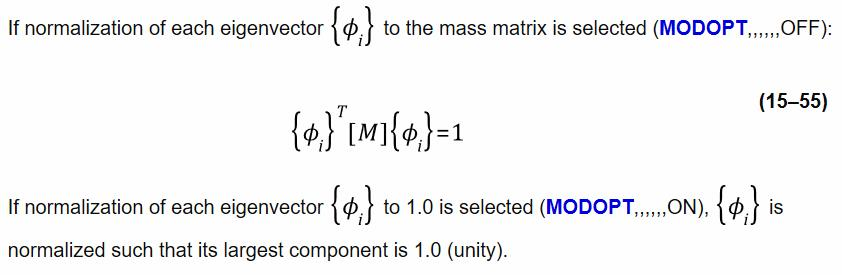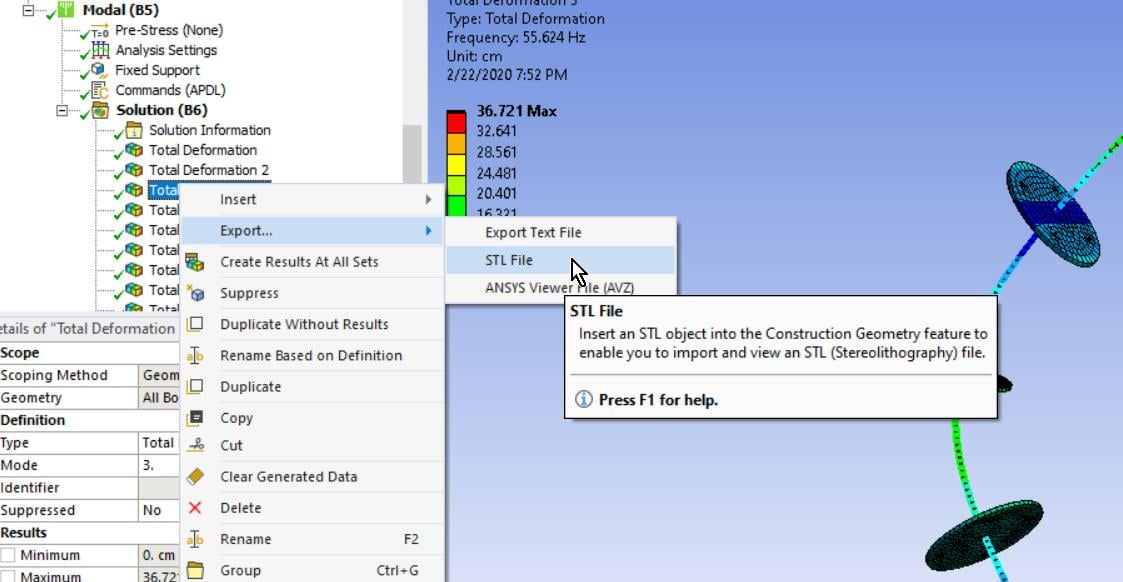# Comparing stresses from modal analysis and static analysis

Hello everyone,

For my master thesis, I use the Mechanical APDL 15.0 with the help of which I would like to carry out a static or modal analysis of a piston rod.

My goal is to get the stresses at the nodes for the elements and modes of the piston rod.

I define a txt. script with the necessary conditions to perform a modal analysis.

ANSYS returns the nodal stresses.

Now I would like to choose an alternative way for getting the stresses (in order to check if the stresses are equal):

a static analysis with defining the shifts as constraints with the "d" command of the respective modes.

If I choose the way of the static analysis, the values for the nodal stresses are different then the nodal stresses I got with the modal analysis.

How can this be?

Is this a constant factor between these two values of the modal analysis and the static analysis that ANSYS always includes in the calculation?

And if so, how is this factor to be identified? How are nodal stresses normalized in ANSYS?

Best regards

Katerina

• Hello Katerina,

Modal analysis computes the shape and frequency of modes and displays a magnitude of displacement and stress that has no meaning for the displacement and stress of a real structure because there is no load applied to the structure in a modal analysis.

The Static Structural solution has an applied load, so the displacements and stresses represent real values. The question for you is what load are you applying, how are you applying the load and what boundary conditions are you using.

Kind regards,

Peter

• edited September 2018
Hello Peter,

I would like to clarify that I already know that the stresses' magnitude has so meaning in modal analysis.
I would just like to know the factor that Ansys is using to normalize the stresses' magnitude.
In other words: How is the stresses' magnitude scaled?
Since the stresses' magnitudes behave should increase linearly or decrease linearly between static and modal analysis at the respective modes there has to be a factor connecting the stresses of static and modal analysis.

Best regards
Katerina
• Hello Katerina,

Both Modal and Static Structural use the same material, which has a Young's modulus, E.

Both Modal and Static Structural compute deformations, which result in strains, which can be multiplied by E to get stress.

So Deformation > Strain > Stress. That is the commonality.

Perhaps that answers part of your question. But you have another part to your question and that is how does ANSYS normalize the deformation in a Modal analysis.

Your statement below has an incorrect assumption.

Since the stresses' magnitudes behave should increase linearly or decrease linearly between static and modal analysis at the respective modes there has to be a factor connecting the stresses of static and modal analysis.

There is no relationship between the deformation in a Static Structural model and the deformation in a Modal analysis because the Static Structural model has a load and the Modal analysis has no load.

If you want to know how ANSYS decides how much deformation to put into the Mode shape for each mode, that is a different question and unrelated to the deformation of a Static Structural model. I don't know the answer to that question. I can use the results of a Modal analysis without knowing this because the magnitude of the deformation has no meaning to me. It is only the shape and frequency that is important.

Kind regards,

Peter

• edited January 2020

Can I know, how the mode shapes are normalized conducting Model Analysis in Ansys Workbench?

• You can choose between two normalizations and I think the mass matrix is the default.• edited January 2020

Thank you peteroznewman for answer. But how to change the option to normalize making largest component to Unity in Mechanical Workbench 19 R1.

• Click on the Modal branch of the outline, click on the Environment tab in the ribbon, click on the Commands button.

The Worksheet will replace the Graphics window where you can type the command shown below, which requests 12 modes, then you can Solve.

`MODOPT,,12,,,,ON`
• edited January 2020

Thank you for reply i will try

• edited February 2020

Hi Peter,

I am a student and working on modal analysis. I want the strain mode shapes from the Ansys workbench. Is it possible to extract them directly from Ansys using export? Another question is I want to do an explicit analysis with sinusoidal and impact excitation to extract the transient response of the cantilever beam. please suggest the analysis to use (Ex: transient structural in ansys).

Kind Regards

Raja Sekhar

• Yes, you can export an STL file of the Mode Shape.Please create a New Discussion for your other question as it does not pertain to this discussion.

• edited March 2020

Hi again,

I've tried to run these two different normalization methods to the same pre-stressed model together with the maxpand. Element stress results are both available and they seems to be in a linear scale relationship. I doubt the stress outputs are based on the model shape displacement fields applied to the structure. If so, then the stress results are not really meaningless, however I'm not sure whether the large deformation is considered since then the structural stiffness is not linear. Can anyone here confirm this?

Regards
Zach

Click on the Modal branch of the outline, click on the Environment tab in the ribbon, click on the Commands button.

The Worksheet will replace the Graphics window where you can type the command shown below, which requests 12 modes, then you can Solve.

`MODOPT,,12,,,,ON`
• Zach,

Modal = Linear analysis

Large Deformation = Nonlinear analysis

Pre-stressed Modal is still a Linear analysis, even if the Static Structural model that computed the pre-stress was a Nonlinear analysis because Large Deflection was turned on.  At the end of that process, the stiffness matrix includes the effect of the pre-stress forces, but the Modal is still a linear analysis of that stiffness and mass matrix.

• edited March 2020

Hi Peter,

Thanks a lot for your answer, but how about the stress? Are the stresses corresponding to the same displacement fields as the normalized mode shapes applied to the pre-stressed structure? I don't know how does ANSYS does the calculation of the stress in a modal analysis. Any comments?

Regards
Zach

• Hi Zach,

Don't look at stress in a Modal analysis, it is not relevant.
Look at stress in a Harmonic Response or Transient Structural analysis where it is relevant.

Regards,
Peter

• edited March 2020

Hi Peter,

I know the stress from the modal analysis is meaningless. However, my wondering is how does ANSYS compute the stresses from a modal analysis? It must be some kind of prescribed disp. applied and it is not totally 'meanlingless'. The followed Harmonic or transient analysis is applicable only if you have known input loads and is not the question here.

Regards
Zach

• The meaningful part of a Modal analysis is the frequency and deformed shape, which can be scaled two ways as explained above.

Stress is always E*Strain in a linear analysis. I don't look at stress results in Modal, that is all I have to say on your question.

• Hi, peter.

first of all. i thank you for all the knowledge i have obtained from you through reading your replies to queries posted almost everywhere here in the forum. i am making my first query here to you.

how is strain calculated from the available deformation? what is the principle of working for ANSYS static strucutural.

i understand from your above reply that the commonality is deformation -> strain -> stress. we know that stress = E*strain. but what is the relation between deformation and strain? is it strain = change in dimension/original dimension?

also i'd be grateful if you could explain or leave a link that explains the working of ansys in the background (from the application of boundary conditions to the solution) what happens when we click on solve and wait till we get the solution.

i generally use static structural analysis and my nonlinearities are currently only geometrical and not material related.

also share some material tips and tricks that are hidden in ANSYS for a static str user that normally goes unnoticed and could make our work easier.

once again, keep up your good work. lots of us are benefitting. :)

• You can click the Ask a Question button on the Structures page to get some answers. The questions above are a bit off-topic of this discussion so I will be brief. Please start a new thread if you have more questions.

Engineering Strain = (change in length)/(original length)

This site has many free courses. Take a look at this series: https://courses.ansys.com/index.php/courses/structural-mechanics-understanding-the-physics/

A good way to learn is to define a question you want to answer and build the model to get that answer. I'm happy to help. Start a new thread if you do that.

• please, Please, how do I find the strain mode shape using ansys workbench?